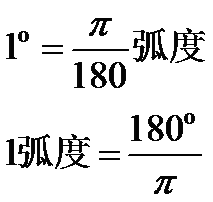# python 三角函数 计算

python中三角函数计算当然就是直接进math库，这没啥说的，导入这个库就行。但是下边计算的时候度这个东西就难搞了，怎么表示？？？？print(math.tan(math.pi/4))    输出：  0.9999999999999999    这次就对了

print(math.atan(1))   输出：  0.7853981633974483  怎么又是一堆乱码

print(math.atan(1)*180/math.pi)  输出： 45.0   这就对了

import math

# π/2 的正弦值
print(math.sin(math.pi/2))
# 1.0

#  1 的反正弦值
print(math.asin(1))
# 1.5707963267948966    π/2

# π 的余弦值
print(math.cos(math.pi))
# -1.0

#  -1 的反余弦值
print(math.acos(-1))
# 3.141592653589793

# 四分之三 π 的正切值
print(math.tan(math.pi*3/4))
# -1.0000000000000002

# 使用 math.degrees(x) 函数查看 四分之一 π 的角度
print(math.degrees(math.pi/4))
# 45.0

# 使用 math.radians(x) 函数查看 135° 对应的弧度制
print(math.radians(135))
# 2.356194490192345
print(math.pi*3/4)
# 2.356194490192345

# math.hypot(x, y) 查看 sqrt(x*x + y*y) 的值
print(math.hypot(3,4))
# 5.0
print(math.hypot(6,8))
# 10.0

print(math.sqrt(6*6 + 8*8))
# 10.0

09-2103-142125
09-191万+
12-03103
06-212296
02-05284
08-288081
05-24
10-26730
04-184499
12-06258
11-0196
12-03189
03-292075
12-04738
©️2020 CSDN 皮肤主题: 大白 设计师:CSDN官方博客